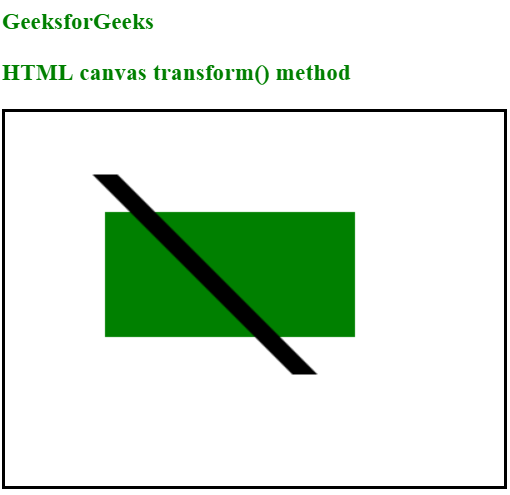# HTML | canvas transform() Method

The transform() method is used to replace the current transformation matrix i.e. each object on the canvas has a current transformation matrix. The transform() method is used to multiply that current transformation matrix with the matrix described below:

```a    c    e
b    d    f
0    0    1```

It simply allows to scale, move, skew and rotate the current context.
Syntax:

`context.transform(a, b, c, d, e, f);`

Parameter Values:

• a denotes Horizontal scaling
• b denotes Horizontal skewing
• c denotes Vertical skewing
• d denotes Vertical scaling
• e denotes Horizontal moving
• f denotes Vertical moving

Example:

 ` ` `<``html``> ` ` `  `<``body``> ` `    ``<``h3` `style``=``"color:green"``>  ` `      ``GeeksforGeeks ` `  `` ` `    ``<``h3` `style``=``"color:green"``> ` `      ``HTML canvas transform() method ` `  `` ` `    ``<``canvas` `id``=``"gfgCanvas"` `            ``width``=``"400"`  `            ``height``=``"300"`  `            ``style``=``"border:2px solid ;"``> ` `    `` ` ` `  `    ``<``script``> ` `        ``var gfg = document.getElementById("gfgCanvas"); ` `        ``var context = gfg.getContext("2d"); ` ` `  `        ``context.fillStyle = "green"; ` `        ``context.fillRect(80, 80, 200, 100) ` ` `  `        ``context.transform(1, 0, 2, 2, 10, 10); ` `        ``context.fillStyle = "black"; ` `        ``context.fillRect(20, 20, 20, 80); ` `    `` ` ` `  ` ` ` `  ` `

Output:Supported Browsers:

• Chrome
• Mozilla Firefox
• Internet Explorer 9.0
• Opera
• Safari

My Personal Notes arrow_drop_upCheck out this Author's contributed articles.

If you like GeeksforGeeks and would like to contribute, you can also write an article using contribute.geeksforgeeks.org or mail your article to contribute@geeksforgeeks.org. See your article appearing on the GeeksforGeeks main page and help other Geeks.

Please Improve this article if you find anything incorrect by clicking on the "Improve Article" button below.

Article Tags :
Practice Tags :

Be the First to upvote.

Please write to us at contribute@geeksforgeeks.org to report any issue with the above content.